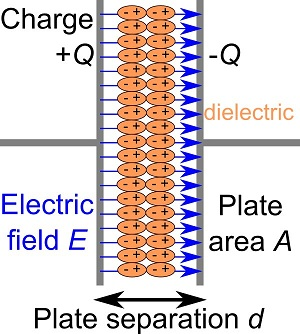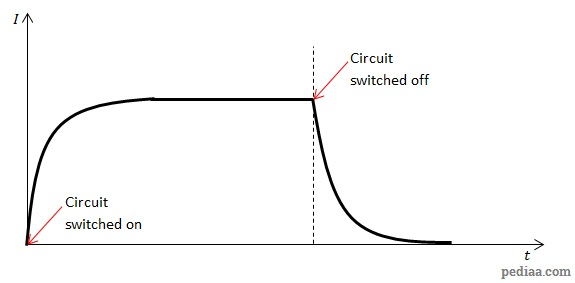# Difference Between Capacitor and Inductor

## Main Difference – Capacitor vs. Inductor

Capacitors and inductors are both circuit components that oppose changes in current in circuits. The main difference between capacitor and inductor is that a capacitor stores energy in the form of an electric field whereas an inductor stores energy in the form of a magnetic field.

## What is a Capacitor

A capacitor is a device that can store energy in the form of an electric field. The simplest form of a capacitor consists of two parallel conducting plates separated by an insulator (a “dielectric” substance) between them.Structure of a capacitor

When a capacitor is connected to an electric circuit, excess charges accumulate on the capacitor’s plates. The two plates acquire the same amounts of opposite charges. As a result, an electric field develops across the plates.

Capacitance$C$ is defined as the ratio of charge$Q$ stored in the plate of a capacitor to the potential difference$V$ across the capacitor.$C=\frac{Q}{V}$

If the parallel plates have a surface area$A$ each, and they are separated by a distance$d$ with a dielectric with a permittivity$\epsilon$ between them, then the capacitance of the plates is given by$C=\epsilon \frac{A}{d}$

The energy$E$ stored in a capacitor with a capacitance$C$ when it has a potential difference$V$ across it is given by:$E=\frac{1}{2}CV^2$

If a capacitor is connected in series with a resistor in a DC circuit, when the circuit is switched on, the current will flow. However, as charges accumulate on capacitors, the potential difference developing across them oppose the potential difference that is driving the current. Since the capacitor’s potential difference builds up, the current decays exponentially and eventually the current flow ceases. If a capacitor is connected to an AC circuit instead, the capacitive reactance causes the current to lead the emf.

## What is an Inductor

An inductor is a device that can store energy in the form of a magnetic field. The simplest form of an inductor consists of a coiled conductor.

When an inductor is connected to an electric circuit, a current flows through the coils in the conductor. Since magnetic fields form around moving charges, a magnetic field forms inside the coil. If the magnetic flux through the coil is given by$\Phi$, and if the coil has$N$ turns and the current flowing around the coil is$I$ then the inductance$L$ is given by:$L=\frac{N\Phi}{I}$

The magnetic energy stored in an inductor with inductance$L$ carrying a current$I$ is given by:$E=\frac{1}{2}LI^2$

If an inductor is connected to a DC circuit in series with a resistor, when the circuit is switched on and the current starts to flow in the coils of the inductor, there is a change in magnetic flux across the coil. According to Faraday’s and Lenz’s laws, an EMF would develop across the inductor which opposes the increasing flow of current. The opposition is stronger when the switch is just turned on, but grows weaker as the rate of change of current reduces. Eventually, a steady current flows in the circuit. If the DC circuit is switched off, as the current through the coils of the inductor falls, there is again a rate of change of magnetic field across the coil and so the inductor should oppose the reduction in current. The figure below shows how these changes in current take place:An inductor in a DC circuit

When an inductor is connected to an AC circuit, the inductive reactance causes the current to lag behind the EMF.

## Difference Between Capacitor and Inductor

### Energy Storage:

Capacitors store energy in the form of an electric field.

Inductors store energy in the form of magnetic fields.

### Characteristics of Capacitor and Inductor:

#### In DC Circuit:

When a capacitor is added in series with a resistor in a DC circuit and the circuit is switched on, the current is initially high but then falls to zero exponentially.

When an inductor is added in series with a resistor in a DC circuit and the circuit is switched on, initially the current is small, but the current increases with time.

#### In AC Circuit:

When a capacitor is added to an AC circuit, it makes the current lead the EMF.

If an inductor is added to an AC circuit, it makes the current lag behind the EMF.

#### Current:

No current passes through a capacitor‘s plates.

However, current passes through the coils in an inductor.

Image Courtesy:

“Schematic of a parallel plate capacitor with a dielectric spacer…” by Papa November (self-made SVG version of Image:Dielectric.png, incorporating Image:Capacitor schematic.svg as its base.) [CC BY-SA 3.0], via Wikimedia Commons

“Electronic component – various small inductors” by me (Photograph) [CC BY-SA 3.0], via Wikimedia Commons